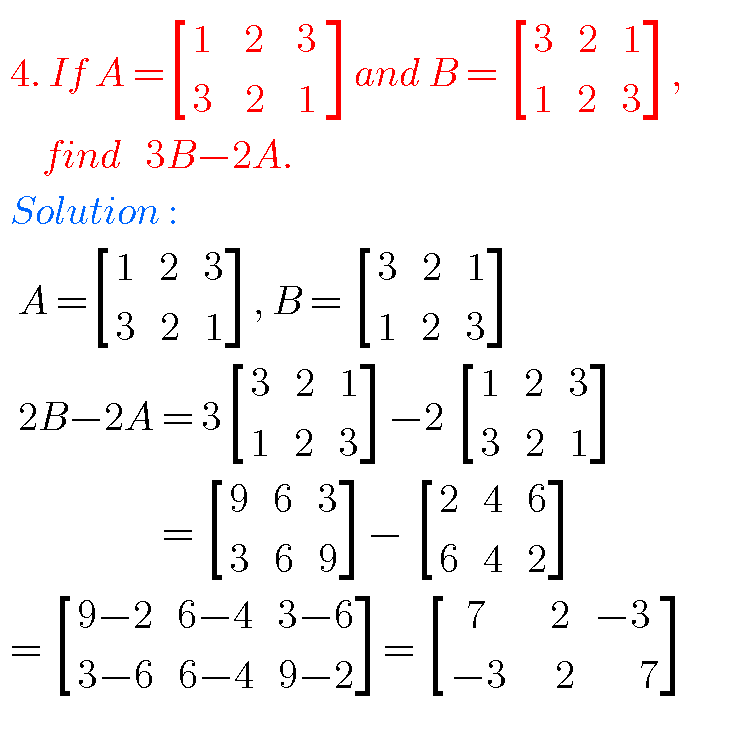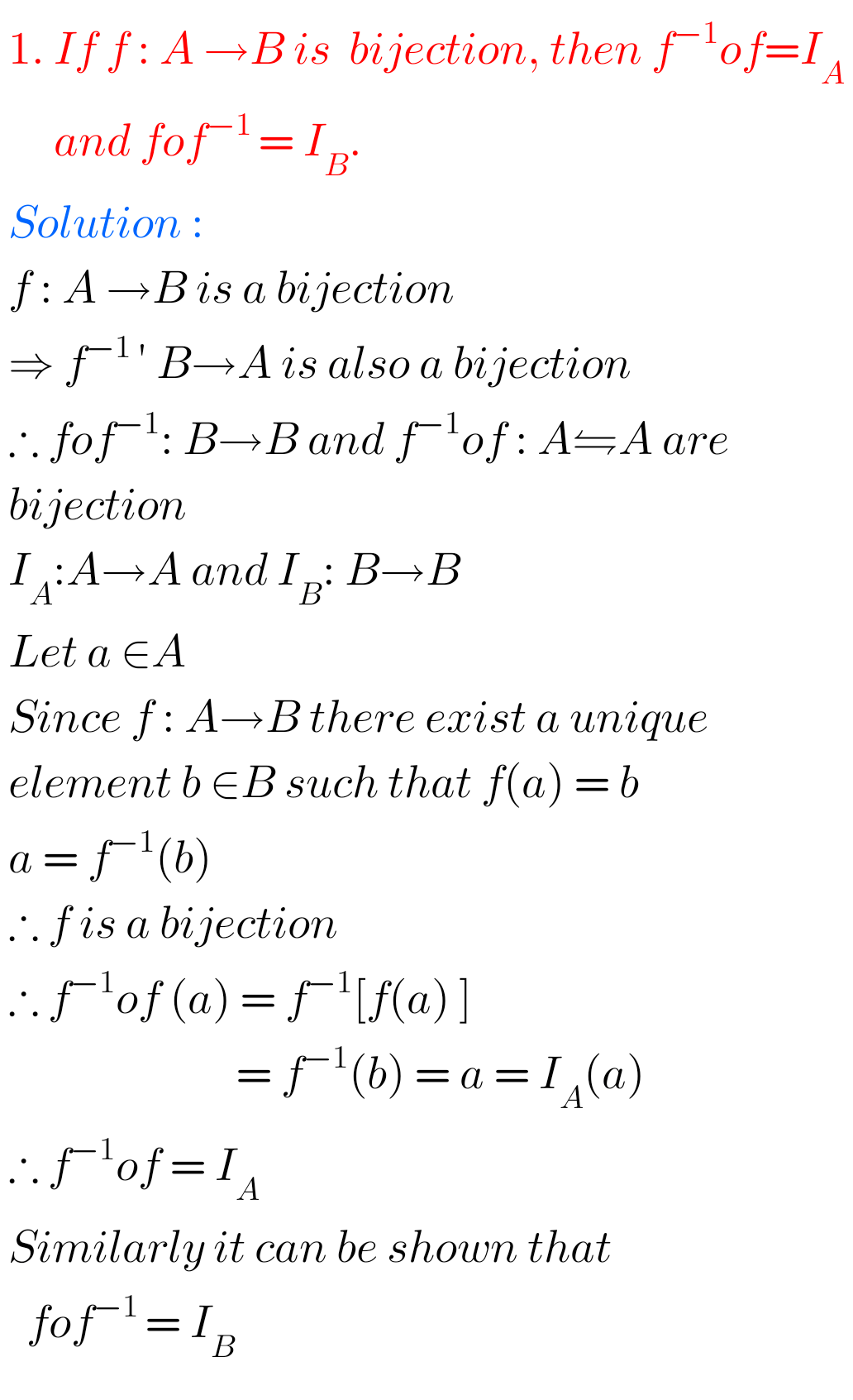# mathematics## Trigonometric Ratios upto Transformations Intermediate Mathematics first year 1A problems with solutions/Trigonometry

INTERMEDIATE MATHEMATICS PROBLEMS WITH SOLUTIONS Solutions for some problems Inter Mathematics first year 1A Trigonometric Ratios upto Transformations. The solutions are very simple to understand. Study the lesson Trigonometric Ratios upto Transformations very well. Textbook exercise solutions links are also given. You can also see Inter Maths 1A textbook solutions Inter Maths 1B textbook solutions …## Product of Vectors,Intermediate first year 1A chapter 5 problems with solutions

PRODUCT OF VECTORS INTERMEDIATE FIRST YEAR 1A CHAPTER 5 PROBLEMS WITH SOLUTIONS Mathematics 1A Intermediate Product of vectors some problems with solutions are given below for examination purpose.  These are very simple to understand. Study the textbook lesson Product of Vectors very well. Observe the example problems and solutions given in the textbook. You can also …## Addition of Vectors,Intermediate first year Mathematics 1A chapter 4 problems with solutions,Junior inter Maths Vector Algebra

ADDITION OF VECTORS, INTERMEDIATE FIRST YEAR MATHEMATICS 1A PROBLEMS WITH SOLUTIONS Intermediate mathematics first year 1A  Addition of vectors. The solutions are very easy to understand. For examination purpose for some problems and solutions are given  Observe the given solutions and try them in your own method You can also see the text book solution …## Matrices solutions,Inter Maths 1a chapter 3 solutions

Matrices solutions, Inter maths 1a chapter 3 solutions Mathematics intermediate first year 1a matrices solutions for some problems for examination purpose. Study the text book lesson Matrices very well. Practice the example problems and solutions given in the textbook. Observe the solutions and try them in your own method. You can also see Inter Maths …## Functions,Intermediate,Solutions

SOLUTIONS OF FUNCTIONS INTERMEDIATE MATHEMATICS 1 A Intermediate maths first year Functions solutions for some problems for examination purpose are given. The solutions are very simple to understand. Study the textbook lesson Functions very well. Observe the example problems and solutions given in the textbook  You can also see the solutions  SSC Maths text book …

## NCERT solutions for class 11th maths chapter 4,Principle of Mathematical Induction

Principle of Mathematical Induction solutions class 11 Ncert CBSE solutions for class 11 Pribciple of Mathematical Induction solutions are given in pdf Principle of Mathematical induction solutions Ncert Mathematical induction solutions for inter Functions solutions for intermediate Properties of triangles solutions for intermediate Trigonometric ratios upto transformations solutions intermediate.  Trigonometric equations solutions intermediate Product of …